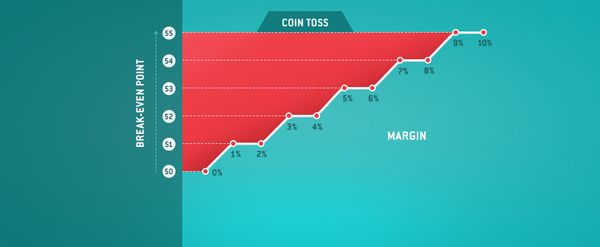# Sports betting profitability and the affect of margins

Do you know what a ‘margin’ is and how crucial it is to your sports betting profitability? The bigger the bookmaker’s margin, the more accurate you have to be with your bets to make a long-term profit. Find out more.##### Margin and Best Odds

In essence the margin – also known as a betting over-round or market percentage – is the additional amount over 100% that the odds on all equally exclusive outcomes of an event imply.

Let’s use a fair die toss as an example. Each outcome (number) has the same probability (1/6) of occurring. Therefore for example, every £1 bet placed on rolling a ‘3’ should return £6 (including your £1 stake).

The decimal odds for this example is 1/probability = 1/(1/6) = 6.00 while its equivalent moneyline odds are +500.

However, a betting company needs to make a profit and therefore would offer odds of 5 rather than 6, which doesn’t fairly reflect the implied probability. Over six die tosses, your number is expected to come up once. Therefore if you staked £6 but received £5, the bookmaker makes £1 in profit.

It is however, important to note that in reality streaks are possible (read the law of large numbers).

In this case, the implied probability per outcome is 20% (1/5). However if you calculate all six outcomes, the sum of probabilities would be 120% (6 outcomes x 20% per outcome). The difference between the 100% and this sum of probabilities is the margin. In this case it is 20%, in favour of the bookmaker. Click here to learn how to calculate a betting margin.

##### So how do margins affect your betting?

Essentially, the higher the bookmaker’s margin is, the bigger impact it will have on your sports betting profitability.

In the example given above, at odds of 5.00, the bettor must make correct guesses of the outcome 20% of the time. Out of 100 guesses, the bettor would need to make 20 correct guesses to break-even.

Therefore for a bookmaker margin of 20%, the bettor would need to correctly predict three extra rolls of the die – as the bettor needs to make 17 correct guesses (1/6 of 100) if there is no margin.

Let’s now examine the break-even point with different margins to highlight how this impacts your sports betting profitability. We used four examples – a coin toss, a roll of the die, an event that has a 90% chance of occurring and an unlikely event that has a 10% chance – with each tested over 100 simulations.

For a coin-toss, the fair odds would be 2.00 on any outcome. In this case bettors would need to make 50 correct guesses to break-even. However, if the margin was 5%, then the odds provided would be 1.904.

In this case, 53 guesses from 100 are needed to break-even. As can be proven mathematically, the graphs bellow all indicate a similar trend, the higher the bookmaker margin, the more correct bets a bettor must make.

##### What have we learnt?

All strategic long-term bettors seek to maximise their returns by obtaining the highest odds possible. The higher the margin, the lower the odds are, and to compensate, an increase in correct guesses by the bettor to obtain break-even is needed.

Essentially this increase is a rounding up of the margin multiplied by the break-even if the margin was zero. For example in a coin toss, the break-even, if the margin was zero, is fifty guesses. When a margin of 3% is applied, the increase is 3% of 50, which is 1.5, but this is rounded up to 2.

Therefore 52 guesses would be required if the margin was 3%. Markets on popular sports are offered as low as 2% at Pinnacle, compared to an industry average of 5% – clearly resulting in more value.

This article identifies how crucial margins are to sports betting profitability. The bigger the margin, the more accurate your bets must be to win in the long run. Bettors choose Pinnacle because the low margins they offer provide a greater chance of sustainable success compared to our competitors.

Dominic Cortis is a lecturer with the Department of Mathematics at The University of Leicester; and an assistant lecturer at The University of Malta. He is an associate actuary and his research focuses on sports analytics as well as financial and betting derivatives.##### MORE: Best E-Sports Betting Sites >>>

Source: pinnacle.com